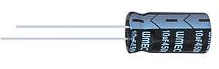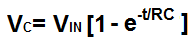﻿ Capacitor Charge (Charging) Calculator ﻿# Capacitor Charge (Charging) CalculatorEnter the Voltage, VIN: μV (microvolts) mS (millivolts) V (volts) Enter the Time, t: μS (microseconds) mS (milliseconds) S (seconds) Enter the Resistance, R: Ω (ohms) KΩ (kilohms) MΩ (megohms) Enter the Capacitance, C: pF (picofarads) nF (nanofarads) mF (millifarads) µF (microfarads) F (farads)

Amount of voltage capacitor has charged to:

The Capacitor Charge/Charging Calculator calculates the voltage that a capacitor with a capacitance, of C, and a resistor, R, in series with it, will charge to after time, t, has elapsed. You can use this calculator to calculate the voltage that the capacitor will have charged to after a time period, of t, has elapsed.In order to calculate the voltage across the capacitor, we must know the voltage, VIN, which supplies voltage to the capacitor, charging it up, , the capacitance, C, of the capacitor, the resistor, R, in series with the capacitor, and the amount of time that has elasped since the charging began. Once we know these, we can calculate the voltage across the capacitor using the formula shown above.

The 4 variables that affect how much the capacitor charges to are the input voltage, VIN, the time, t, that has passed since the charging process starts, the resistance, R, of the resistor, and the capacitance, C, of the capacitor.

The input voltage, VIN is critical to what the capacitor will charge to. The greater the input voltage, the more the capacitor will charge up to. Capacitors will only charge to the voltage that they are exposed to. So if a capacitor is exposed to a larger voltage, it will charge to a greater value. If it is charged by a smaller voltage, it will charge to a smaller voltage value. The input voltage has a direct relationship with VC.

The time, t, is very important during the charging process. The more time that elapses, the more time the capacitor has to charge. The shorter the time, the shorter period of the time the capacitor has to charge. Therefore, the greater the value of t, the greater the charge voltage, VC, the capacitor will be. The smaller the time, t, the smalle VC.

The resistance, R, of the resistor determines how fast or slow the capacitor charges. If the resistance is greater, the charging process will be slower, since resistance slows down current. If the resistance is lower, there is less resistance, so the capacitor can charge quicker. Thus the larger the resistance, the smaller VC will be. The smaller the resistance, the greater VC will be.

The capacitance, C, of the capacitor determines how long it will take for the capacitor to charge. If the capacitance is larger, it will take a longer time to charge. This is because there is more ability or room for the capacitor to store charge. Conversely, the smaller the capacitance, the quicker it takes for the capacitor to fill, because there is less room for storing charge. So the greater the capacitance, the smaller VC. The smaller the capacitance, the greater VC.

The value of the equation result gives VC, which is the voltage that is across the capacitor. The resultant voltage is in unit volts (V).

Capacitor charging is useful because in many circuits capacitors are charged with DC voltage for many different uses and functions. Capacitors can act as voltage reservoirs in circuits which release voltage to a circuit if the main power source is disconnected. Capacitors are also charged with DC voltage for other purposes. In flash cameras, capacitors are charged with charge, when winding the camera over and over, as in mechanical cameras. This charges the capacitor. When taking a picture, all of this charge from this capacitor is dumped all at once, allowing for the flash, for the picture to be taken.

Related Resources

﻿